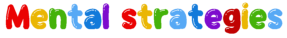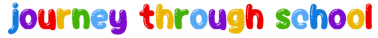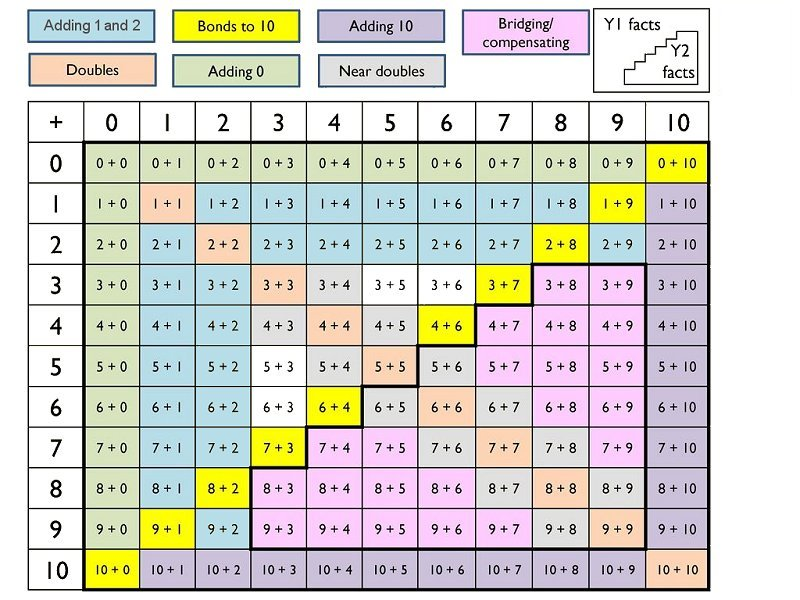# Beckstone Primary School

Bridging communities | Promoting learning and excellence | Shaping the future.

Eadie Street, Harrington, Workington, Cumbria CA14 5PX
01946 830423 | wb@beckstone.cumbria.sch.uk |The grid below shows KS1 number bonds used to secure number knowledge##### Year 1
###### Rapid recall

Y1 facts on grid tested and recorded termly – including associated subtraction facts

###### Mental strategies

+ 0 + 1 and + 2 (and –) to any number up to 20 (Just 0, 1, or 2 more/less)

+/- 10 to any 1 digit number including zero:10 + 7 = 17

Notice Number bonds to 10

Doubling and halving: double facts and halves to 5 + 5 (and 10 + 10)

Near number bonds to add two one digit numbers:
"7 + 2 = 9 because 7 + 3 = 10 so it's just one less" or "8 + 3 mist be 11 because 8 + 2 = 10"

Near double facts e.g. "3 + 4 = 7 because double 3 is 6 so it's just one more".

Partitioning: Use number facts to add TO + O: "24 + 3 . . . I know that 3 + 4 = 7 so 20 + 7 = 27"

Adjusting: 'make ten' supported by models and images e.g. 8 + 6 = 8 + 2 + 4

##### Year 2
###### Rapid recall

Y1 and 2 facts on grid tested and recorded termly – including associated subtraction facts

2, 5 and 10 times table multiplication and division facts

###### Mental strategies

Number bonds to 10 and near number bonds to add two or three single digit numbers

Spot doubles and near doubles to add two or three single digit numbers

Use number bonds to 20 and near number bonds to 20 to add 2 numbers

+ 10 to any 2 digit number (support with models, images and hundred square)

Partitioning: Calculations with whole numbers which do not involve crossing place value boundaries e.g.
23 + 45= ? by 40 + 5 +20 + 3 or 40 + 23 + 5

Counting on or back in tens and ones to add or subtract – flexibility with number line

Adjusting: 'make ten' supported by models and images e.g. 8 + 6 = 8 + 2 + 4

+/- multiples of 10 where the answer is between 0 and 100 (e.g. 70 + 30 = 100, 20 + 40 = 60)

Doubling and halving: Derives doubles and halves of multiples of 10 up to 100

Doubling and halving: Find the doubles to 100 using partitioning and halves of any even number to 100

##### Year 3
###### Rapid recall

3, 4 and 8 times table and associated division facts

Multiply 2 digit number by 10

+/- multiples of 10 where the answer is between 0 and 100 (e.g. 70 + 30 = 100, 20 + 40 = 60)

Doubles and halves of multiples of 10 up to 100

###### Mental strategies

Counting on or back in fives from any multiple of 5– e.g. 35+15=? by counting on in steps of 5 from 35

Counting on or back in hundreds from any number e.g. 570 + 300= ? by counting on in hundreds from 570

Partitioning: Calculations with whole numbers which involves crossing place value boundaries e.g. 42 – 28= ? by 42 – 2 – 20 - 6

Adjusting multiples of 10 e.g. 38 + 68= ? by 38 + 70 – 2 or 45 – 29 = 45 – 30 + 1

Adjusting: 'make ten' progressing to multiples of ten e.g 28 + 13 = 30 + 11

Near doubles to numbers under 20 e.g. 18 + 16 is double 18 and subtract 2 or double 16 and add 2

Near doubles to multiples of 10 e.g. 60 + 70 is double 60 and add 10 or double 70 and subtract 10

Doubling and halving: Find the doubles and halves of any two-digit number and any multiple of 10 or 100– e.g. half 680 or double 73

Doubling and halving: Multiply and divide by 4 by doubling/halving twice and 8 by doubling/halving again. – e.g. 34 x 4 = 34 x 2 x 2.

##### Year 4
###### Rapid recall

All multiplication and division facts up to 12 x 12

+/- multiples of 10 beyond 100 e.g. 50 + 60 = 110

Half of any even number to 100

Multiply and 2 or 3 digit number by ten

###### Mental strategies

Counting on or back in tenths and/or hundredths- e.g. 3.2 + 0.6 = ? by counting on in tenths. 1.7 + 0.55=? by counting on in tenths and hundredths – flexibility with a number line

Adjusting multiples of 10 or 100 e.g. 138 + 69= ? by 138 + 70 – 1 or 299 – 48 = 300 – 48 – 1

Adjusting 'make ten' progressing to 3 digit numbers e.g. 128 + 32 = 130 + 30

Partitioning: Calculations with decimal numbers not crossing place value boundaries then crossing boundaries.
E.g. 3.2 + 2.1 progressing to 3.7 + 6.8

Near doubles to 100 e.g. 75 + 76 is double 76 and subtract 1 or double 75 and add 1

Near doubles to numbers under 20 e.g. 18 + 16 is double 18 and subtract 2 or double 16 and add 2

Doubling and halving: Find the doubles and halves of any number up to 1,000 by partitioning

##### Year 5
###### Rapid recall

+/- multiples of 1000

Multiply and divide any number by 10 and 100

Halves of any number to 100 (e.g. half of 22 = 11, half of 51 = 25.5)

Squares of all numbers up to 12

Cubes of 2, 3, 4 and 5

###### Mental strategies

Adjusting multiples with decimals e.g 2 ½ + 1 ¾ by 2½ + 2 – ¼ or 5.7 + 3.9 by 5.7 + 4.0 – 0.1

Decimal near doubles to whole numbers e.g. 2.5 + 2.6 is double 2.5 add 0.1 or double 2.6 subtract 0.1.

Doubling and halving: Find the doubles and halves of any number up to 10,000 by partitioning – e.g. half of 32,202 by halving 3,000, 2000, 200 and 2

Doubling and halving: Multiply by 50 by multiplying by 100 and halving e.g. 8 x 50= 8 x 100 divided by 2

Doubling and halving: Double and half decimal number with up to one decimal place by portioning – e.g. half of 8.4 by halving 8 and halving 0.4

##### Year 6
###### Rapid recall

Multiplication of multiples of 10 and 100 based on known facts (e.g. 40 x 40 = 1,600);

###### Mental strategies

Adjusting multiples with decimals e.g 2 ½ + 1 ¾ by 2½ + 2 – ¼ or 5.7 + 3.9 by 5.7 + 4.0 – 0.1

Decimal near doubles to whole numbers e.g. 2.5 + 2.6 is double 2.5 add 0.1 or double 2.6 subtract 0.1.

Doubling and halving: Find the doubles and halves of any number up to 10,000 by partitioning – e.g. half of 32,202 by halving 3,000, 2000, 200 and 2

Doubling and halving: Multiply by 50 by multiplying by 100 and halving e.g. 8 50= 8 x 100 divided by 2

Doubling and halving: Double and half decimal number with up to one decimal place by portioning – e.g. half of 8.4 by halving 8 and halving 0.4